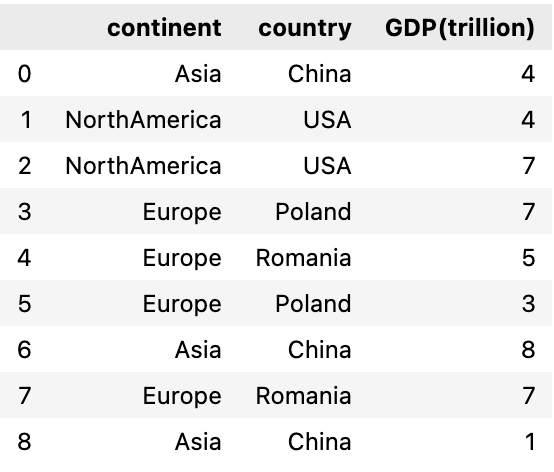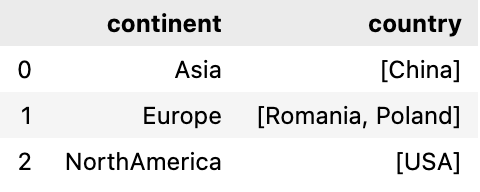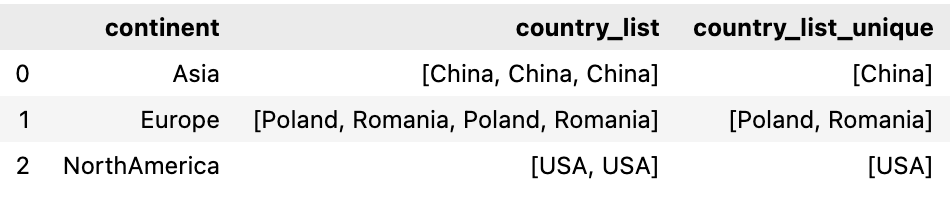# pandas groupby list of values

In this post, we will learn how to create list of values in a pandas groupby.

We will first create a dataframe of 4 columns , first column is continent, second is country and third & fourth column represents their GDP value in trillion and Member of G20 group respectively. These are fake numbers and doesn’t represent their real GDP worth.

Once this dataframe is created then we will group the dataframe by continent and list all countries in each group

## Create a dataframe

Let’s create a dataframe with all the four columns: continent, country, GDP(trillion) and Member_G20

For the third column GDP(trillion), I’m using numpy randint function to create random numbers for all these countries

``````import pandas as pd
import numpy as np

df = pd.DataFrame({'continent' : ['Asia','NorthAmerica','NorthAmerica','Europe','Europe', 'Europe','Asia', 'Europe', 'Asia'],
'country' : ['China', 'USA', 'Canada', 'Poland', 'Romania', 'Italy', 'India', 'Germany', 'Russia'],
'GDP(trillion)' : np.random.randint(1, 9 , 9)})
``````### Pandas groupby and list of values in a column

So we will first group by continent and then list all the countries under a continent

we will use apply and list function that will list group-wise and combine the results together.

While apply is a very flexible method, its downside is that using it can be quite a bit slower than using more specific methods

``````df.groupby(['continent'])['country'].apply(list)
``````

Output:

``````continent
Asia                         [China, China, China]
Europe          [Poland, Romania, Poland, Romania]
NorthAmerica                            [USA, USA]
Name: country, dtype: object
``````

Let’s explore other ways to list the values

we will use groupby agg function to aggregate this list of countries operation over the specified axis

``````df.groupby('continent')['country'].agg(list)
``````

Next, if you want to return a datafame with the list of values as a column then just reset the index as shown below

``````df.groupby('continent')['country'].apply(list).reset_index()
``````Now if you want to rename this column of list of values then pass name parameter in the reset_index function as your new column name

``````df.groupby('continent')['country'].apply(list).reset_index(name='country_list')
``````

Output### Pandas groupby and list of unique values

The list of values may contain duplicates and in order to get unique values we will use set method for this

``````df.groupby('continent')['country'].agg(lambda x: list(set(x))).reset_index()
``````Alternatively, we can also pass the set or unique func in aggregate function to get the unique list of values

``````df.groupby('continent')['country'].agg(set).reset_index()

or

df.groupby('continent')['country'].agg('unique').reset_index()
``````

finally, if we want to see both the list of values and it’s unique value in the dataframe then we can use groupby named aggregation

``````df.groupby('continent').agg(country_list=('country',list),
country_list_unique=('country','unique')).reset_index()
``````Tags:

Categories:

Updated: